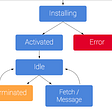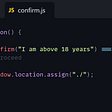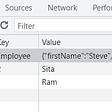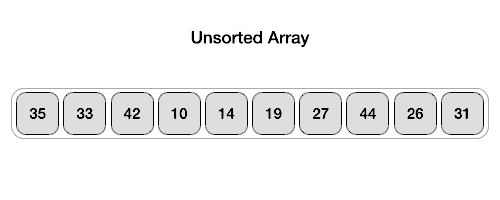# How to create a BST:

`class Node {   constructor (val) {      this.val = val;       this.left = null;       this.right = null;   }}`
`class BST {    constructor (val) {     this.root = null    }}`
`class BST {    constructor (val) {      this.root = null    }   insert (val) {         }}`

# How to insert into a BST

1. Create a variable newNode that takes in a new Node from the previous class.
2. If there is no root, then given the value of new node, assign the new node to be the root.
3. Create a variable called current to be equal to the root.
4. While true, if the current value is equal to the value given in the parameter, return undefined.
5. If the value is less than the current value, and if the current.left is null, assign the current.left equal to newNode. Once this is assigned, reassign current to be current.left (aka null)
6. If the value is greater than the current value, and if the current.right is null, assign the current.right = to the newNode. Once this is assigned, reassign the current to be current.right (aka null)
`class BST {   constructor() {   this.root = null   }   insert(val){   let newNode = new Node(val)   if (!this.root) {   this.root = newNode   return this;  }   let current = this.root;   while (true){   if (current.val === val) return undefined;     if (val < current.val) {         if (current.left === null) {            current.left = newNode            return this;         }      current = current.left      } else {         if (current.right === null) {         current.right = newNode          return this;        }     current = current.right     }   }}`
`let newTree = new BST()`
`newTree.insert(5)newTree.insert(6)newTree.insert(7)newTree.insert(3)`
`    5  /  \3      6         \           7`

# LeetCode 653

`const traverseDFS = (tree, k) => {    let hash = new Set() // o (1)    let stack = [tree]    while (stack.length > 0) {   // o (n)       let current = stack.pop()              if (hash.has(current.val)) {         return true       }         hash.add(k - current.val)       if (current.left) stack.push(current.left)        if (current.right) stack.push(current.right)   }   return false }`

--

--

--

## More from Crystal Villanueva

Flatiron student, word nerd and otter enthusiast

Love podcasts or audiobooks? Learn on the go with our new app.

## Service Workers in Web Development## One Minor (Major) Glitch in Running a Node App with NGINX as a Reverse Proxy## How To Start A Project with bootstrap 5 and webpack 5 (Step by step)## Using JavaScript’s Inbuilt Confirm() Function## Introduction to Window localStorage## How To Mock API In React## Compose Simple State## Crystal Villanueva

Flatiron student, word nerd and otter enthusiast

## File IO in Java 8 — Part I## 4-in-1 Priority First Search in Python: BFS, DFS, Prim’s, and Dijkstra’s algorithms## A programmer’s experience of Pair Programming## Different Sorting Algorithms